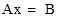# NMath User's Guide

19.3 Sparse Matrix Factorizations (.NET, C#, CSharp, VB, Visual Basic, F#)

NMath provides classes for computing and storing factorizations of general sparse matrices. Instances of the factorization classes calculate solutions to the equationwhere A is a sparse matrix and B is a single vector, or multiple vectors.

Once a factorization is constructed, it can be reused to solve for different right-hand sides.

Factorization Classes

The factorization classes associated with each general sparse matrix type are shown in Table 16.

 Matrix Classes Factorization Classes FloatCsrSparseMatrix FloatSparseFact FloatSymCsrSparseMatrix FloatSparseSymFact FloatSparseSymPDFact FloatComplexCsrSparseMatrix FloatComplexSparseFact FloatHermCsrSparseMatrix FloatSparseHermFact FloatSparseHermPDFact DoubleCsrSparseMatrix DoubleSparseFact DoubleSymCsrSparseMatrix DoubleSparseSymFact DoubleSparseSymPDFact DoubleComplexCsrSparseMatrix DoubleComplexSparseFact DoubleHermCsrSparseMatrix DoubleSparseHermFact DoubleSparseHermPDFact

Note that there are two factorization classes for symmetric and Hermitian types: one for indefinite matrices, and one for positive definite (PD) matrices.

SparseMatrixFact is the base class for sparse matrix factorizations, and is parameterized on the type, T, of values stored in the vector.

Creating Factorizations

You can create an instance of a factorization class by supplying the constructor with a matrix to factor. The following code creates a symmetric sparse matrix from the given data, then factors the matrix:

Code Example – C# sparse matrix factorization

```var sA = new DoubleSymCsrSparseMatrix( sparseData);
```
```var fact = new DoubleSparseSymFact( sA );
```

Code Example – VB sparse matrix factorization

```Dim SA As New DoubleSymCsrSparseMatrix(SparseData)
```
```Dim Fact As New DoubleSparseSymFact(SA)
```

You can also use an existing instance to factor other matrices with the provided Factor() method. Thus, if sB is another DoubleSymCsrSparseMatrix:

Code Example – C# sparse matrix factorization

```fact.Factor( sB );
```

Code Example – VB sparse matrix factorization

```Fact.Factor(SB)
```

The read-only ErrorStatus property gets an Error enumerated value. For example:

Code Example – C# sparse matrix factorization

```if ( fact.ErrorStatus == DoubleSparseSymFact.Error.NoError )
```
```{
```
```   // Do something here...
```
```}
```

Code Example – VB sparse matrix factorization

```If Fact.ErrorStatus = DoubleSparseSymFact.Error.NoError Then
```
```  ' Do something here...
```
```End If
```

Using Factorizations

Once a factorization is constructed from a matrix, it can be used to solve for different right hand sides. For instance, this code solves for one right-hand side:

Code Example – C# sparse matrix factorization

```var b = new DoubleVector( 8, 1 );
```
```DoubleVector x = fact.Solve( b );
```

Code Example – VB sparse matrix factorization

```Dim B As New DoubleVector(8, 1)
```
```Dim X As DoubleVector = Fact.Solve(B)
```

Similarly, you can use the Solve() method to solve for multiple right-hand sides. This code solves for 3 right-hand sides:

Code Example – C# sparse matrix factorization

```int nrhs = 3;
```
```var B = new DoubleMatrix( 8, nrhs, new RandGenBeta() );
```
```DoubleMatrix X = fact.Solve( B );
```

Code Example – sparse matrix factorization

```Dim NRHS As Integer = 3
```
```Dim B As New DoubleMatrix(8, NRHS, New RandGenBeta())
```
```Dim X As DoubleMatrix = Fact.Solve(B)
```

The right-hand sides are the columns of matrix B, and the corresponding solutions are the columns of matrix X.

NOTE—Be sure to check the ErrorStatus property on the factorization before calling Solve() to confirm that the factorization is valid.

Top

Top